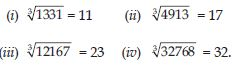# NCERT Solutions for Class 8 Math Chapter 7 - Cubes and Cube Roots

##### Question 1:

Find the one’s digit of the cube of each of the following numbers.
(i) 3331 (ii) 8888 (iii) 149
(iv) 1005 (v) 1024 (vi) 77
(vii) 5022 (viii) 53

As we know that, the cube of a number ending in 0, 1, 4, 5, 6 and 9 ends in 0, 1, 4, 5, 6 and 9 respectively. However, the cube of a number ending in 2 ends in 8 and vice-versa. Similarly, the cube of a number ending in 3 or 7 ends in 7 or 3 respectively. Thus, by looking at the unit digit of a given number, we can determine the unit’s digit of its cube. Now, unit’s digit of the cube of each of the asked numbers is as follows: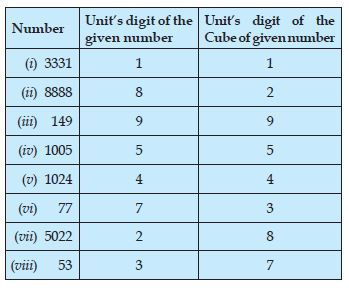##### Question 2:

Express the following number as the sum of odd numbers using the above pattern.
63

63 = 216 = 31 + 33 + 35 + 37 + 39 + 41

##### Question 3:

Express the following number as the sum of odd numbers using the above pattern.
83

83 = 512 = 57 + 59 + 61 + 63 + 65 + 67 + 69 + 71

##### Question 4:

Express the following number as the sum of odd numbers using the above pattern.
73

73 = 343 = 43 + 45 + 47 + 49 + 51 + 53 + 55

##### Question 5:

Consider the following pattern:
23 - 13 = 1 + 2 x 1 x 3
33 - 23 = 1 + 3 x 2 x 3
43 - 33 = 1 + 4 x 3 x 3
Using the above pattern, find the value of the following:
73 - 63

73 – 63
According to the given pattern:
73 – 63 = 1 + 7 x 6 x 3

##### Question 6:

Consider the following pattern: 23 – 13 = 1 + 2 x 1 x 3
33 – 23 = 1 + 3 x 2 x 3
43 – 33 = 1 + 4 x 3 x 3
Using the above pattern, find the value of the
following:
123 – 113

123 – 113
According to the given pattern:
123 – 113 = 1 + 12 x 11 x 3

##### Question 7:

Consider the following pattern: 23 – 13 = 1 + 2 x 1 x 3
33 – 23 = 1 + 3 x 2 x 3
43 – 33 = 1 + 4 x 3 x 3
Using the above pattern, find the value of the following:
203 – 193

203 – 193
According to the given pattern:
203 – 193 = 1 + 20 x 19 x 3

##### Question 8:

Consider the following pattern:
23 – 13 = 1 + 2 x 1 x 3
33 – 23 = 1 + 3 x 2 x 3
43 – 33 = 1 + 4 x 3 x 3
Using the above pattern, find the value of the following:
513 – 503

513 – 503
According to the given pattern:
513 – 503 = 1 + 51 x 50 x 3

##### Question 9:

Check whether 400 is perfect cube or not.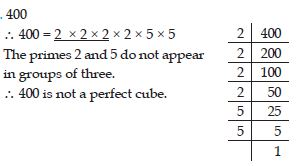##### Question 10:

Check whether 3375 is perfect cube or not.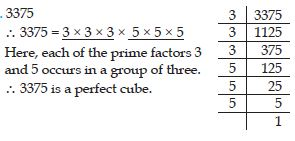##### Question 11:

Check whether 8000 is perfect cube or not.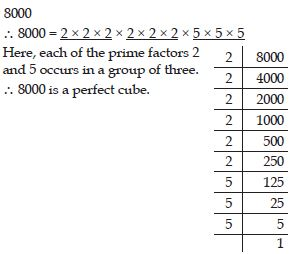##### Question 12:

Check whether 15625 is perfect cube or not.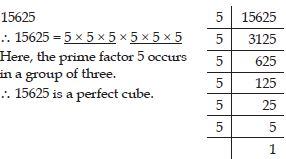##### Question 13:

Check whether 9000 is perfect cube or not.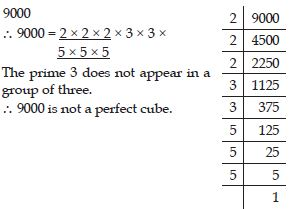##### Question 14:

Check whether 6859 is perfect cube or not.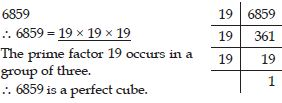##### Question 15:

Check whether 2025 is perfect cube or not.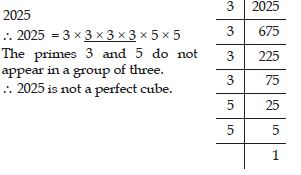##### Question 16:

Check whether 10648 is perfect cube or not.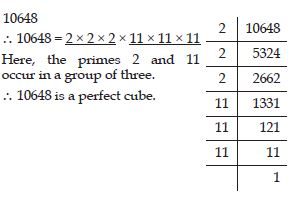##### Question 17:

Check whether 2700 is perfect cube or not.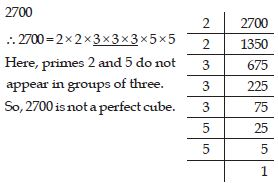##### Question 18:

Check whether 16000 is perfect cube or not.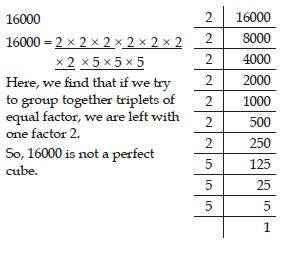##### Question 19:

Check whether 64000 is perfect cube or not.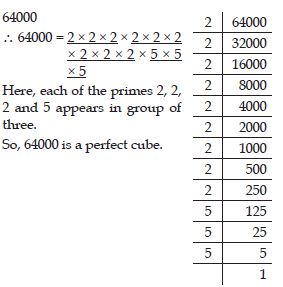##### Question 20:

Check whether 900 is perfect cube or not.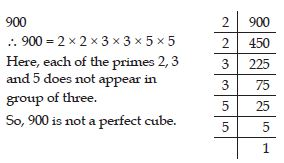##### Question 21:

Check whether 125000 is perfect cube or not.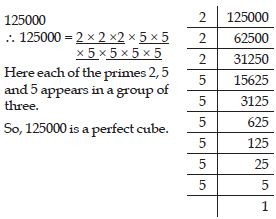##### Question 22:

Check whether 36000 is perfect cube or not.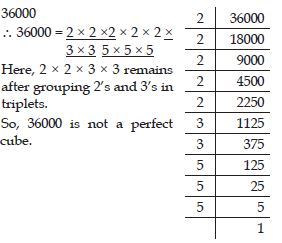##### Question 23:

Check whether 21600 is perfect cube or not.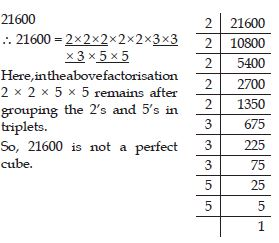##### Question 24:

Check whether 10000 is perfect cube or not.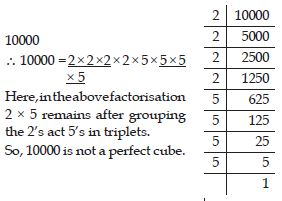##### Question 25:

Check whether 27000000 is perfect cube or not.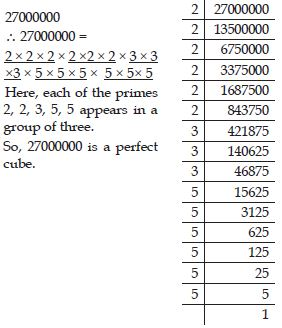##### Question 26:

Check whether 1000 is perfect cube or not.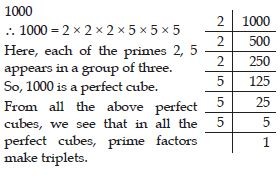##### Question 27:

Check whether 216 is perfect cube or not.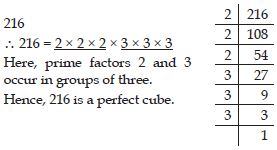##### Question 28:

Check whether 128 is perfect cube or not.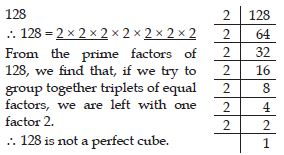##### Question 29:

Check whether 1000 is perfect cube or not.##### Question 30:

Check whether 100 is perfect cube or not.##### Question 31:

Check whether 46656 is perfect cube or not.##### Question 32:

Find the smallest number by which 243 must be multiplied to obtain a perfect cube.

243 = 3 × 3 × 3 × 3 × 3
We note that, prime factor 3 appears in a group of 3. Further, we are left with two more factors 3.
So, if we multiply 3 × 3 by 3 i.e., then in the product 3 will appear in one more group of three and the product will be a perfect cube. i.e.,
243 × 3 = 3 × 3 × 3 × 3 × 3 × 3 = 729
which is a perfect cube.
Hence, the smallest whole number by which 243 should be multiplied to make a perfect cube is 3.

##### Question 33:

Find the smallest number by which 256 must be multiplied to obtain a perfect cube.

256
256 = 2 × 2 × 2 × 2 × 2 × 2 × 2 × 2
We note that, prime factor appears in groups of 3. Further, we are left with two more factors 2.
To make it a perfect cube, we need one more 2.
In that case:
256 × 2 = 2 × 2 × 2 × 2 × 2 × 2 × 2 × 2 × 2 = 512
which is a perfect cube.
Hence, the smallest whole number by which 256 should be multiplied to make it a perfect cube is 2.

##### Question 34:

Find the smallest number by which 72 must be multiplied to obtain a perfect cube.

72
72 = 2 × 2 × 2 × 3 × 3
We note that, prime factor 2 appears in groups of 3. Further, we are left with two more factors 3.
To make it a perfect cube, we need one more 3.
In that case:
72 × 3 = 2 × 2 × 2 × 3 × 3 × 3 = 216
which is a perfect cube.
Hence, the smallest whole number by which 72 should be multiplied to make it a perfect cube is 3.

##### Question 35:

Find the smallest number by which 675 must be multiplied to obtain a perfect cube.

675
$\therefore$ 675 = 3 × 3 × 3 × 5 × 5
We note that, in prime factorisation of 675, prime factor 3 appears in a group of 3. Further, we are left with two more factors 5.
To make it a perfect cube, we need one more 5.
In that case:
675 × 5 = 3 × 3 × 3 × 5 × 5 × 5 = 3375
which is a perfect cube.
Hence, the smallest whole number by which 675 should be multiplied to make it a perfect cube is 5.

##### Question 36:

Find the smallest number by which 100 must be multiplied to obtain a perfect cube.

100
100 = 2 × 2 × 5 × 5
We note that, in the prime factorisation of 100, the prime factors 2 and 5 are not in groups of 3.
$\therefore$ We have, one more 2 and one more 5 to make them in groups of 3.
In that case:
100 × 2 × 5 = 2 × 2 × 2 × 5 × 5 × 5 = 1000
which is a perfect cube.
Hence, the smallest whole number by which 100 should be multiplied to make it a perfect cube is 10.

##### Question 37:

Find the smallest number by which 81 must be divided to obtain a perfect cube.

81
$\therefore$ 81 = 3 × 3 × 3 × 3
The prime factor 3 does not appear in a group of three.
$\therefore$ 81 is not a perfect cube.
In the prime factorisation of 81, one factor 3 remains ungrouped.
So, 81 is not a perfect cube.
If we divide 81 by 3, then the prime factorisation of the quotient becomes 81 ÷ 3 = 3 × 3 × 3 = 27 which is a perfect cube.
Hence, the smallest number by which 81 should be divided to make it a perfect cube is 3.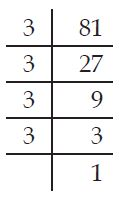##### Question 38:

Find the smallest number by which 128 must be divided to obtain a perfect cube.

128
$\therefore$ 128 = 2 × 2 × 2 × 2 × 2 × 2 × 2
In the prime factorisation of 128, one factor 2 remains ungrouped.
So, 128 is not a perfect cube.
If we divide 128 by 2, then the prime factorisation of the quotient becomes 128 ÷ 2 = 2 × 2 × 2 × 2 × 2 × 2 = 64
which is a perfect cube.
Hence, the smallest number by which 128
should be divided to make it perfect cube is 2.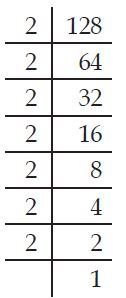##### Question 39:

Find the smallest number by which 135 must be divided to obtain a perfect cube.

135
$\therefore$ 135 = 3 × 3 × 3 × 5
In the prime factorisation of 135, the factor 5 remains ungrouped.
So, 135 is not a perfect cube.
If we divide 135 by 5, then the prime factorisation of the quotient becomes 135 ÷ 5 = 3 × 3 × 3 = 27
which is a perfect cube.
Hence, the smallest number by which 135 should be divided to make it a perfect cube is 5.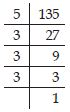##### Question 40:

Find the smallest number by which 192 must be divided to obtain a perfect cube.192
$\therefore$ 192 = 2 × 2 × 2 × 2 × 2 × 2 × 3
In the prime factorisation of 192, the factor 3 remains ungrouped.
So, 192 is not a perfect cube.
If we divide 192 by 3, then the prime factorisation of the quotient becomes
192 ÷ 3 = 2 × 2 × 2 × 2× 2× 2 = 64
which is a perfect cube.
Hence, the smallest number by which 192
should be divided to make it a perfect cube is 3.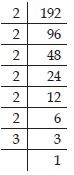192
$\therefore$ 192 = 2 × 2 × 2 × 2 × 2 × 2 × 3
In the prime factorisation of 192, the factor 3 remains ungrouped.
So, 192 is not a perfect cube.
If we divide 192 by 3, then the prime factorisation of the quotient becomes
192 ÷ 3 = 2 × 2 × 2 × 2× 2× 2 = 64
which is a perfect cube.
Hence, the smallest number by which 192
should be divided to make it a perfect cube is 3.##### Question 41:

Find the smallest number by which 704 must be divided to obtain a perfect cube.

704
$\therefore$ 704 = 2 × 2 × 2 × 2 × 2 × 2 × 11
In the prime factorisation of 704, the factor 11 remains ungrouped.
So, 704 is not a perfect cube.
If we divide 704 by 11, then the prime factorisation of the quotient becomes 704 ÷ 11 = 2 × 2 × 2 × 2 × 2 × 2
= 64
which is a perfect cube.
Hence, the smallest number by which 704 should be divided to make it a perfect cube is 11.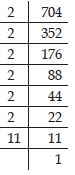##### Question 42:

Parikshit makes a cuboid of plasticine of sides 5 cm, 2 cm, 5 cm. How many such cuboids will he need to form a cube?

The sides of cuboid are 5 cm, 2 cm and 5 cm.
Volume of the plastic in = 2 × 5 × 5 cm3
Here, the prime factors of 2 and 5 are not in groups of three.
$\therefore$ We have to multiply by 2 × 2 × 5 i.e., by 20 to make it a perfect cube.

##### Question 43:

Find the cube root of 64 by prime factorisation method.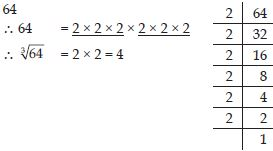##### Question 44:

Find the cube root of 512 by prime factorisation method.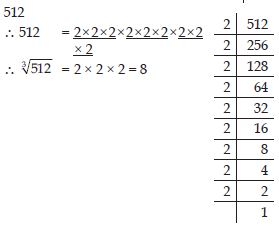##### Question 45:

Find the cube root of 10648 by prime factorisation method.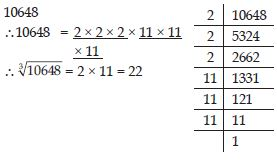##### Question 46:

Find the cube root of 27000 by prime factorisation method.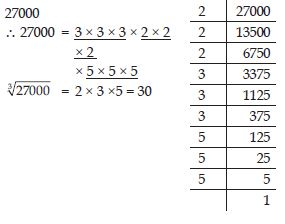##### Question 47:

Find the cube root of 15625 by prime factorisation method.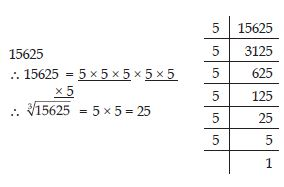##### Question 48:

Find the cube root of 13824 by prime factorisation method.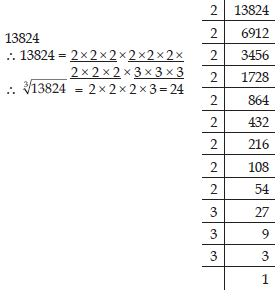##### Question 49:

Find the cube root of 110592 by prime factorisation method.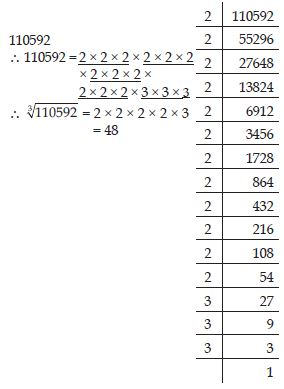##### Question 50:

Find the cube root of 46656 by prime factorisation method.##### Question 51:

Find the cube root of 175616 by prime factorisation method.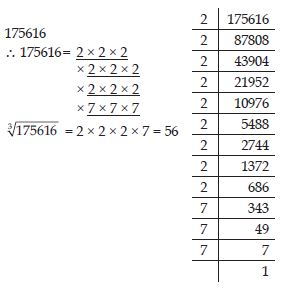##### Question 52:

Find the cube root of 91125 by prime factorisation method.##### Question 53:

Cube of any odd number is even.

1. TRUE
2. FALSE

FALSE

##### Question 54:

A perfect cube does not end with two zeros.

1. TRUE
2. FALSE

TRUE

##### Question 55:

If square of a number ends with 5, then its cube ends with 25.

1. TRUE
2. FALSE

TRUE

##### Question 56:

There is no perfect cube which ends with 8

1. TRUE
2. FALSE

FALSE

##### Question 57:

The cube of two digit number may be a three digit number.

1. TRUE
2. FALSE

FALSE

##### Question 58:

The cube of a two digit number may have seven or more digits.

1. TRUE
2. FALSE

FALSE

##### Question 59:

The cube of a single digit number may be a single digit number.

1. TRUE
2. FALSE